# The Power of Pandas: PythonPandas Basics### Pandas is a Python package providing fast, flexible, and expressive data structures designed to make working with “relational” or “labeled” data both easy and intuitive. It aims to be the fundamental high-level building block for doing practical, real world data analysis in Python. Additionally, it has the broader goal of becoming the most powerful and flexible open source data analysis / manipulation tool available in any language. It is already well on its way toward this goal.

Pandas is a high-level data manipulation tool developed by Wes McKinney. It is built on the Numpy package and its key data structure is called the DataFrame. DataFrames allow you to store and manipulate tabular data in rows of observations and columns of variables.pandas is well suited for many different kinds of data: -Tabular data with heterogeneously-typed columns, as in an SQL table or Excel spreadsheet -Ordered and unordered (not necessarily fixed-frequency) time series data. ・ Arbitrary matrix data (homogeneously typed or heterogeneous) with row and column labels ・ Any other form of observational / statistical data sets. The data actually need not be labeled at all to be placed into a pandas data structure

Here are just a few of the things that pandas does well: ・ Easy handling of missing data (represented as NaN) in floating point as well as non-floating point data -Size mutability: columns can be inserted and deleted from DataFrame and higher dimensional objects -Automatic and explicit data alignment: objects can be explicitly aligned to a set of labels, or the user can simply ignore the labels and let Series, DataFrame, etc. Automatically align the data for you in computations ・ Powerful, flexible group by functionality to perform split-apply-combine operations on data sets, for both aggregating and transforming data -Make it easy to convert ragged, differently-indexed data in other Python and NumPy data structures into DataFrame objects -Intelligent label-based slicing, fancy indexing, and subsetting of large data sets ・ Intuitive merging and joining data sets ・ Flexible reshaping and pivoting of data sets ・ Hierarchical labeling of axes (possible to have multiple labels per tick) Robust IO tools for loading data from flat files (CSV and delimited), Excel files, databases, and saving / loading data from the ultrafast HDF5 format -Time series-specific functionality: date range generation and frequency conversion, moving window statistics, date shifting and lagging.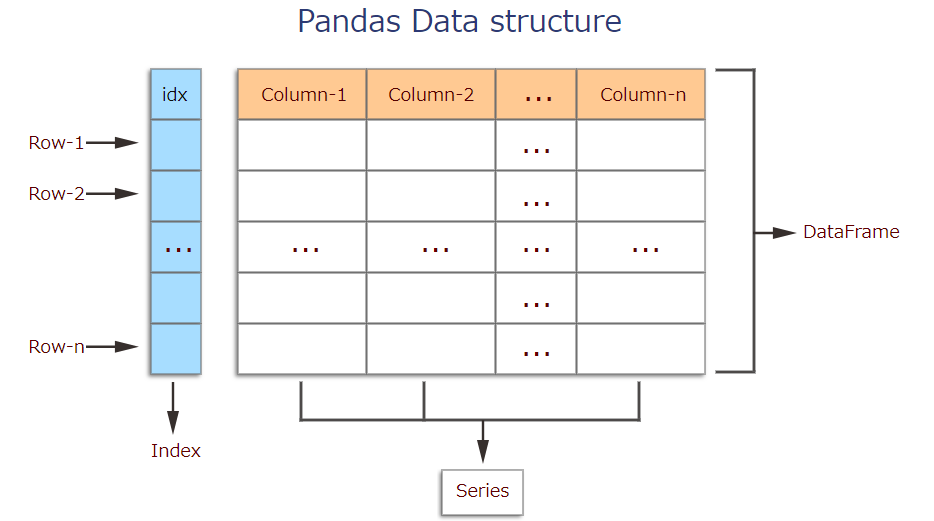To load the pandas package and start working with it, import the package.

``````In : import pandas as pd
``````

■Creating data The two primary data structures of pandas, Series (1-dimensional) and DataFrame (2-dimensional).Each column in a DataFrame is a Series.

** ・ DataFrame ** A DataFrame is a table. It contains an array of individual entries, each of which has a certain value. Each entry corresponds to a row (or record) and a column. For example, consider the following simple DataFrame:

``````In : pd.DataFrame({'Yes': [50, 21], 'No': [131, 2]})
``````

Out :

Yes No
0 50 131
1 21 2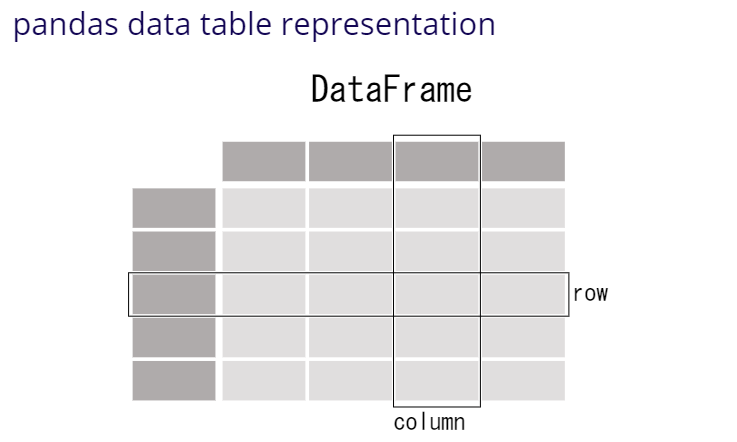DataFrame entries are not limited to integers. For instance, here's a DataFrame whose values are strings:

``````In : pd.DataFrame({'Bob': ['I liked it.', 'It was awful.'], 'Sue': ['Pretty good.', 'Bland.']})
``````

Out :

Bob Sue
0 I liked it. Pretty good.
1 It was awful. Bland.

There are several ways to create a DataFrame. One way is to use a dictionary. For example: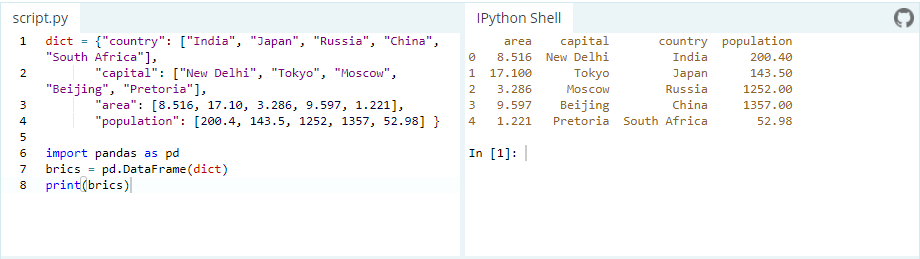** ・ Series ** A Series, by contrast, is a sequence of data values. If a DataFrame is a table, a Series is a list. And in fact you can create one with nothing more than a list: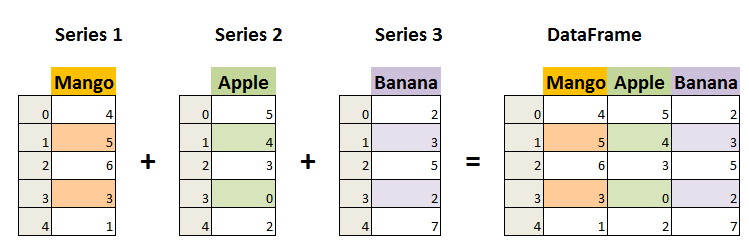``````In : pd.Series([1, 2, 3, 4, 5])
``````
``````Out :
0    1
1    2
2    3
3    4
4    5
dtype: int64
``````

■Reading data files Another way to create a DataFrame is by importing a csv file using Pandas. Data can be stored in any of a number of different forms and formats. By far the most basic of these is the humble CSV file. Now, the csv cars.csv is stored and can be imported using pd.read_csv: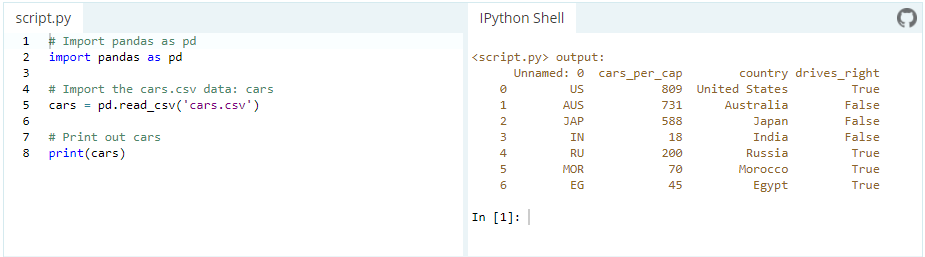or we can examine the contents of the resultant DataFrame using the head() command, which grabs the first five rows:

``````In : pd.head()
``````

■ Other Useful Tricks ** ・ Get the current working directory **

``````In : import os
In : os.getcwd()
``````

** ・ Check how many rows and columns present in the data ** (o/p -> no. of rows, no. of columns)

``````In : pd.shape
Out : (2200, 15)
``````

** ・ Rename the columns **

``````In : pd_new = pd.rename(colums = {'Amount.Requested': 'Amount.Requested_NEW'})
``````

** ・ Write a dataframe in csv or excel **

``````df.to_csv("filename.csv", index = False)
df.to_excel("filename.xlsx", index = False)
``````

There are two ways to handle the situation where we do not want the index to be stored in csv file.

1. you can use index=False while saving your dataframe to csv file.
``````df.to_csv("file_name.csv", index=False)
``````

2 . Or you can save your dataframe as it is with an index, and while reading you just drop the column unnamed 0 containing your previous index.

``````df.to_csv("file_name.csv")
df_new = pd.read_csv("file_name.csv").drop(['unnamed 0'],axis=1)
``````

here is the cheat-sheet for pandas. https://pandas.pydata.org/Pandas_Cheat_Sheet.pdf

Enjoy the Power of Pandas and I hope you found it helpful. Thank you for spending the time to read this article. See you in next topic. :grinning: :grinning: